# thetatickformat

Specify theta-axis tick label format

## Syntax

``thetatickformat(fmt)``
``thetatickformat(pax,___)``
``tfmt = thetatickformat``
``tfmt = thetatickformat(pax)``

## Description

example

````thetatickformat(fmt)` sets the format for the theta-axis tick labels. For example, specify `fmt` as `'usd'` to display the labels in U.S. dollars.```

example

````thetatickformat(pax,___)` uses the axes specified by `ax` instead of the current axes. Specify `pax` as the first input argument.```
````tfmt = thetatickformat` returns the format style used for theta-axis tick labels of the current axes. Depending on the type of labels along the theta-axis, `tfmt` is a character vector of a numeric format.```
````tfmt = thetatickformat(pax)` returns the format style used for the axes specified by `pax` instead of the current axes.```

## Examples

collapse all

Create a polar plot. Display the tick labels along the theta-axis as degree values.

```th = linspace(0,2*pi); r = 2*th; polarplot(th,r) thetatickformat('degrees')```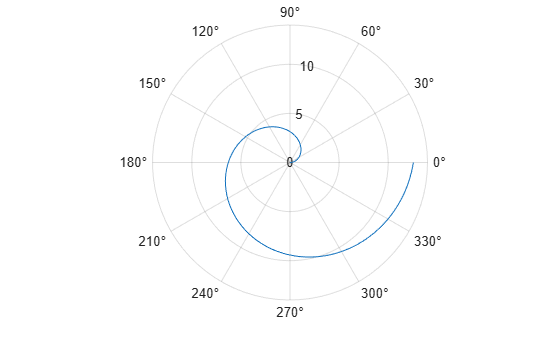Create a polar plot. Display the tick labels along the theta-axis with the text "deg" after each value.

```th = linspace(0,2*pi); r = th.^2; polarplot(th,r) thetatickformat('%g deg')```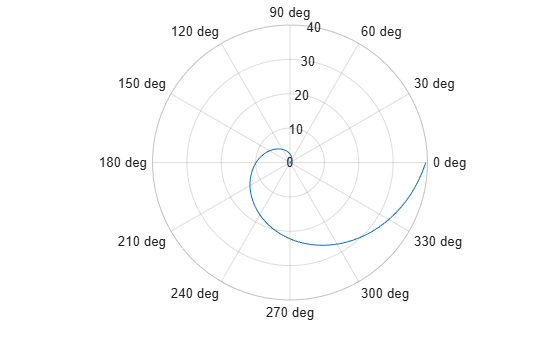Display the theta-axis tick labels with two decimal places. Control the decimal places by passing `thetatickformat` a character vector of a numeric format that uses fixed-point notation for the conversion character and a precision value of 2.

```theta = 0:0.01:2*pi; rho = sin(2*theta).*cos(2*theta); polarplot(theta,rho) thetatickformat('%.2f')```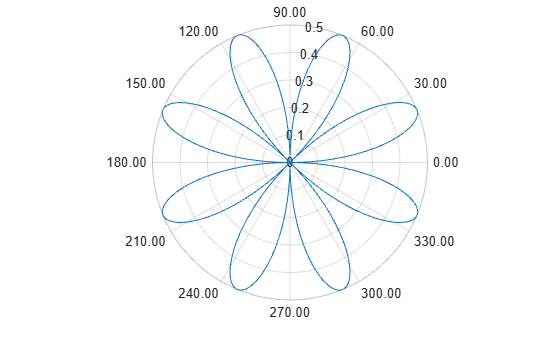Create a polar plot and assign the polar axes object to the variable `pax`. Ensure that `thetatickformat` affects the polar axes you just created by passing `pax` as the first input argument to the function.

```polarplot(1:10,'->') pax = gca; thetatickformat(pax,'degrees')```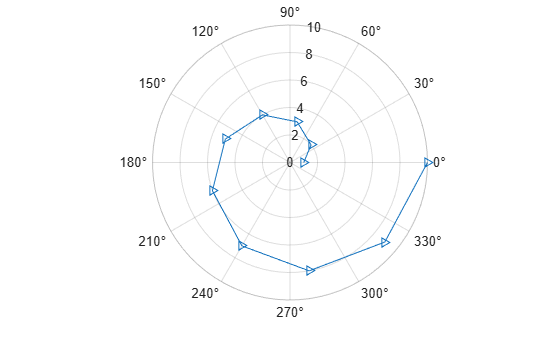## Input Arguments

collapse all

Format for numeric tick labels, specified as a character vector or string. You can specify one of the formats listed in this table. Alternatively, you can specify a custom format.

Predefined FormatDescription
`'usd'`

U.S. dollars. This option is equivalent using `'\$%,.2f'`. If the labels use scientific notation, this option sets the exponent value to 0.

`'eur'`

Euro. This option is equivalent to using `'\x20AC%,.2f'` with an exponent value of 0.

`'gbp'`

British pound. This option is equivalent to using `'\x00A3%,.2f'` with an exponent value of 0.

`'jpy'`

Japanese yen. This option is equivalent to using `'\x00A5%,d'` with an exponent value of 0.

`'degrees'`

Display degree symbol after values. This option is equivalent to using `'%g\x00B0'` with the default exponent value.

`'percentage'`

Display percent sign after values. This option is equivalent to using `'%g%%'` with the default exponent value.

`'auto'`

Default format of `'%g'` with the default exponent value.

Example: `thetatickformat('usd')`

#### Custom Numeric Format

You can specify a custom numeric format by creating a character vector or string containing identifiers.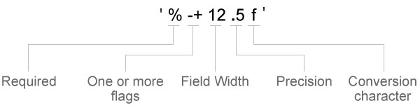Identifiers are optional, except the percent sign and conversion character. Construct the format in this order:

• One or more flags — Options such as adding a plus sign before positive values. For a full list of options, see the table of Optional Flags.

• Field width — Minimum number of characters to print in the tick label. Specify the field width as an integer value. If the number of significant digits in the tick value is smaller than the field width, then the label is padded with spaces.

• Precision — Number of digits to the right of the decimal point or the number of significant digits, depending on the conversion character. Specify the precision as an integer value.

• Conversion character — Value type. For a full list of options, see the table of Conversion Characters. If you specify a conversion that does not fit the data, then MATLAB® overrides the specified conversion, and uses `%e`.

Also, you can specify literal text at the beginning or end of the format. To print a single quotation mark, use `''`. To print a percent character, use `%%`.

Example: `thetatickformat('%.2f')` displays the values using fixed-point notation with two decimal places.

Example: `thetatickformat('\$%.2f')` displays a dollar sign before each value.

Example: ```thetatickformat('%.2f million')``` displays `million` after each value.

Optional Flags

IdentifierDescriptionExample of Numeric Format
`,`Display commas every three digits, such as `'1,000'`.`'%,4.4g'`
`+`Print the sign character (+) for positive values, such as `'+100'`.`'%+4.4g'`
`0`Pad the field width with leading zeros instead of spaces, such as `'0100'`.`'%04.4g'`
`–`Left-justify, which pads the end of the value with spaces instead of the beginning. For example, if the field width is `4`, then this flag formats the label as `'100 '` instead of `' 100'`.`'%-4.4g'`
`#`

For the `%f`, `%e`, and `%g` conversion characters, print the decimal point even when the precision is 0, such as `'100.'`. For `%g`, do not remove trailing zeros.

`'%#4.4g'`

Conversion Characters

IdentifierDescriptionExample
`d` or `i`Signed integer with base 10. The precision value indicates the number of significant digits. `'%.4d'` displays π as `0003`.
`f`Fixed-point notation. The precision value indicates the number of decimal places.`'%.4f'` displays π as `3.1416`.
`e`Exponential notation. The precision value indicates the number of decimal places.`'%.4e'` displays π as `3.1416x100`.
`g`The more compact version of `e` or `f`, with no trailing zeros. The precision value indicates the maximum number of decimal places.`'%.4g'` displays π as `3.1416`.

Polar axes, or an array of polar axes. If you do not specify this argument, then `thetatickformat` modifies the current axes (provided that the current axes is a polar axes object).

## Algorithms

The `thetatickformat` function sets and queries the `TickLabelFormat` property of the ruler object associated with the theta-axis.

## Version History

Introduced in R2016b